# Conic Sections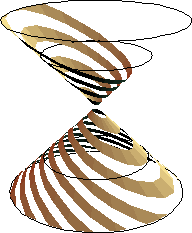Intersections of parallel planes and a double cone, forming ellipses, parabolas, and hyperbolas respectively. graphics code

## History

Conic sections are among the oldest curves, and is a oldest math subject studied systematically and thoroughly. The conics seems to have been discovered by Menaechmus (a Greek, ~375 BC – 325 BC), tutor to Alexander the Great. They were conceived in a attempt to solve the three famous problems of trisecting the angle, duplicating the cube, and squaring the circle. The conics were first defined as the intersection of: a right circular cone of varying vertex angle; a plane perpendicular to a element of the cone. (An element of a cone is any line that makes up the cone) Depending the angle is less than, equal to, or greater than 90 degrees, we get ellipse, parabola, or hyperbola respectively. Appollonius (~262 BC to 190 BC) (known as The Great Geometer) consolidated and extended previous results of conics into a monograph Conic Sections, consisting of eight books with 487 propositions. Quote from Morris Kline: “As a achievement it [Appollonius’ Conic Sections] is so monumental that it practically closed the subject to later thinkers, at least from the purely geometrical standpoint.” Book VIII of Conic Sections is lost to us. Appollonius’ Conic Sections and Euclid's Elements may represent the quintessence of Greek mathematics.

Appollonius was the first to base the theory of all three conics on sections of one circular cone, right or oblique. He is also the one to give the name ellipse, parabola, and hyperbola. A brief explanation of the naming can be found in Howard Eves, An Introduction to the History of Math. 6th ed. page 172. (also see J H Conway's newsgroup message at conicsEtynomogy.txt.)

In Renaissance, Kepler's law of planetary motion, Descarte and Fermat's coordinate geometry, and the beginning of projective geometry started by Desargues, La Hire, Pascal pushed conics to a high level. Many later mathematicians have also made contribution to conics, espcially in the development of projective geometry where conics are fundamental objects as circles in Greek geometry. Among the contributors, we may find Newton, Dandelin, Gergonne, Poncelet, Brianchon, Dupin, Chasles, and Steiner. Conic sections is a rich classic topic that has spurred many developments in the history of mathematics.

## Description

Hyperbola, ellipse, and parabola are together known as conic sections, or just conics. So called because they are the intersection of a right circular cone and a plane.

Conics can be defined as follows. Given a line d and a point F not on d, conics is the locus of points P such that: distance[P,F]/distance[P,d] == e, where e is a given constant. F is called the focus of the conic, d the directrix, and e the eccentricity. If 0 < e < 1, the conics is a ellipse. If e == 1, it is a parabola, if e > 1, it is a hyperbola and has two branches.Conic sections with eccentricities {.2, .4, .6, .8, 1, 1.5, 2, 2.5, 3}. Their focus is at the origin. The directrix is the white line x==1. Conics Family

## Formula

Let the essentricity be e. Let the point F be the Origin. Let the directrix be a vertical line x=1. The polar formula is then:

r == e/(1 +e*Cos[θ])

In Cartesian coordinate, let {x,y} be the point P. We have distance[P,F]/distance[P,d]==e. Using coordinates this becomes Sqrt[(x-0)^2+(y-0)^2]/Sqrt[(x-1)^2+(y-y)^2]==e. Move the square root to one side and square both sides (the radicand is positive since it's sum of sqares), then simplify we have the Cartesian equation:

```(-e^2) + 2*e^2*x + (1 - e^2)*x^2 + y^2 == 0
```

By shifting to the right (replace x by x-(-e^2/(-1+e^2)) ), we can transform this equation to the standard form given for ellipses and hyperbola x^2/a^2 + y^2/b^2 == 1. conics_eq_derive.nb.zip

Any second degree polynomial: a*x^2+2*h*x*y+b*y^2+2*g*x+2*f*y+c==0, where at least one of a,b,h is not 0, is a conic section.

The distance k from the focus to directrix only effect the curve's scaling. The essentricity e changes the curve's shape.

## Properties

### Sections of a Cone and Dandelin Sphere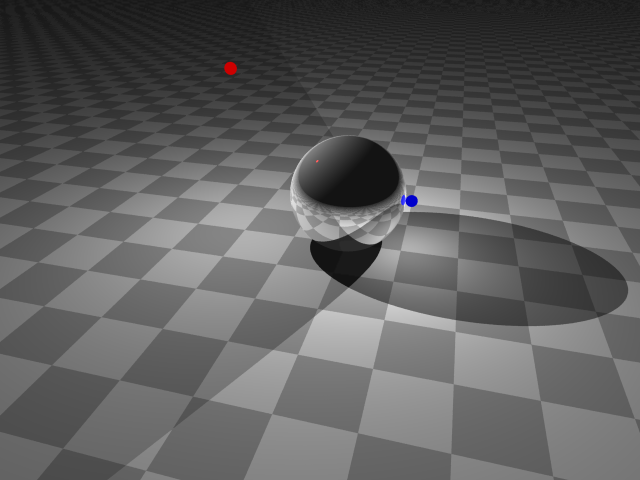Red light source above the ball cast a shadow of ellipse, the blue light source below the height of the ball cast a shadow of hyperbola. The focus of both curve, is the place where the ball touches the floor. Since the two curves shares a focus and axis, their intersections are orthogonal. (source: ball_shadow.pov)

Conic section is the intersection of a right (or oblique) circular cone and a plane. This can be seen as the shadow of a ball placed on a table. The shadow cast by a light source above the ball is a ellipse. If the light source is in a plane parallel to the table that passes the top of the ball, a parabola is formed. Lower the light source, then you get one branch of a hyperbola. The point where the ball touches the table is the focus of the conics. We can think of the light source as the vertex of a cone. The light source projects through a circle on the ball to form a right circular cone. The table is then the cutting plane. More generally, the projection of a circle on any plane forms a conic section. Shadows in the shape of conics are often seen on the wall of a nearby lamp with circular openings in its lampshade.

Let α be the angle between the axis of a right circular cone and its element (the generating lines) Let θ be the (smaller) angle formed by the axis of the cone and the cutting plane. Let d be the distance from the vertex of the cone to the cutting plane.

The relation of α and θ defines the shape of the curve. d affects the scaling or size of the curve, except when d == 0 we get a degenerate conics. The magnitude of d is proportional to the size of the curve. We have the following cases:

• If θ < α, hyperbola; and if d == 0, two non-parallel lines.
• If θ == α, parabola; and if d == 0, a line.
• If θ > α, ellipse; and if d == 0, a point.

Dandelin sphere is a sphere of certain size and position inscribed inside the cone. Dandelin sphere relate many properties of the conics to the cone. It is named after its discoverer Germinal Pierre Dandelin (1822).

1. Inscribe a sphere inside the cone and the cutting plane. Such sphere will be tangent to the cutting plane at a point and tangent to the cone along a circle. For the case of hyperbola and ellipse, there are two such spheres.
2. The point where the sphere touches the cutting plane is the focus of the conics.
3. The directrix of the conics is the intersection of the cutting plane and the plane of the osculating circle.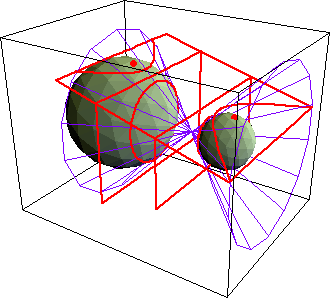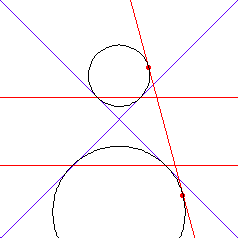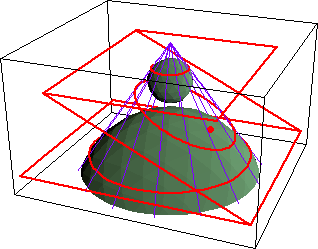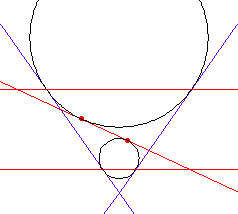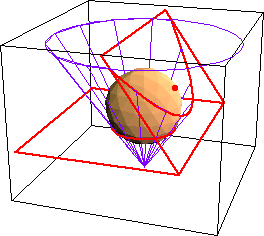Proof: We are going to show that any section of a right circular cone has the proprety distance[P,F] == e distance[P,D], where P is any point on the intersection, F is a fixed point on the cutting plane, D is a fixed line on the cutting plane, and e is a constant, thus by definition it is a conic section. The proof works for all three cases of conics and may take some persistance to work through.

1. Inscribe a sphere that is tangent to: the cone along a circle C; the cutting plane at a point F. Let P be any point on the conics. We shall see that F is a focus of the conics and that the corresponding directrix is the line D in which the cutting plane intersects the plane of circle C.
2. Let Q be the point that is the intersection of: The line through P that is parallel to the axis of the cone; The plane of C.
3. Let A be the point where the line joining P to the vertex of the cone intersects C.
4. Let line PD be the perpendicular from P to line D. Then distance[P,A] and distance[P,F], being tangents to the sphere from a common point P, have the same length:

PA == PF.

From right triangle PQA, we have PQ == PA Cos[α].

From right triangle PQD, we have PQ == PD Cos[θ].

Combining the above three equations and simplify, we get
PA Cos[α] == PD Cos[θ]
PF Cos[α] == PD Cos[θ]
PF == Cos[θ]/Cos[α] PD
End of proof.

Mathematica Notebooks: (does not need each other to run.)

### Ellipse seen as Circle

Any ellipse will appear to be a circle from centain view points. In other words, a ellipse will project into a circle at certain projection point. The set of all such points is a hyperbola, shaped and positioned so that its vertexes is located at the ellipse's foci, and foci is on the ellipse's vertexes, and the plane it resides is orthogonal to the ellipse's. The figures illustrate three ellipses with eccentricities 0.3, 0.7, and 0.9. The corresponding hyperbolas's eccentricities are 1/.3, 1/.7, and 1/.9 respectively.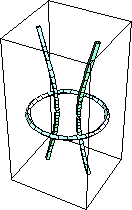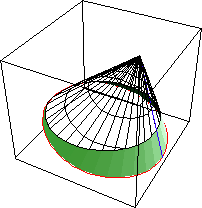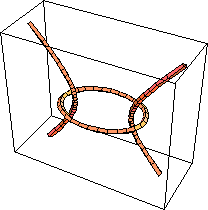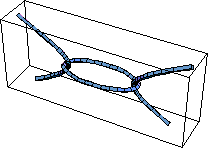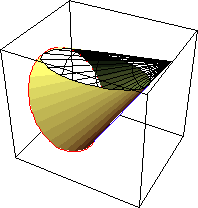Codes for above graphics

The figure below is a middle cross secton of the cone. The red line is the side view of the given ellipse (e==.7) with vertexes at v1, v2 and focus at f1, f2. v0 is the vertex of the cone.Moving v0

v0, v1, and v2 together forms the cone. v0 is the projection point, or eye position, looking towards w. v1, p and v2, q forms the circle we perceive.

We see that as v0 moves on the hyperbola, different cones are generated but the plane v1, v2 will always cut the cone to form the same ellipse. This tells us the relationship of parameters α, θ, and d for a given ellipse.

### Second Degree Curves

The curve of any second degree polynomial equation “A x^2 + B x y + C y^2 + D x + E y + F == 0” is a conics, or degenerate conics (two parallel lines, a point, or no graph). This is one amazing result in mathematics. In the following table, we give a example of each case.

Possible CasesExample
Circle
`x^2 + y^2 == 4`
Parabola
`y^2 == 9 x`
Ellipse
`4 x^2 + 9 y^2 == 36`
Hyperbola
`x^2 - y^2 == 1`
One Line
`x^2 == 0`
Intersecting Lines
`(x - 1) (y + 1) == 0`
Parallel Lines
`(x - 1) (x - 2) == 0`
A Point
`x^2 + y^2 == 0`
No Graph
`x^2 == -1`

### 5-Point Conics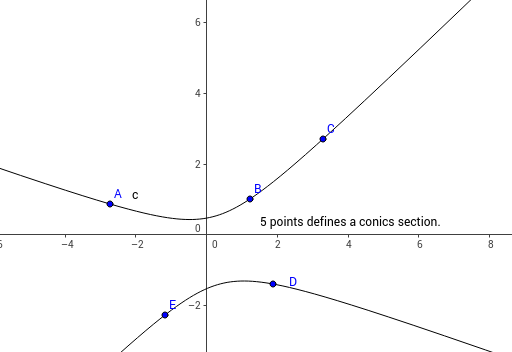5 points defines a conic section uniquely. 5-Point Conics 5 Points Conics Tangent Construction

### Intersection of Circles

The intersections of circles centered at F1 and F2 whose radiuses are arithmetic sequence {r, r+c, r+2 c, …}, are points on hyperbolas and ellipses with foci F1 and F2. This can be easily seen by looking at the definition of hyperbola and ellipse as the difference or sum of distances from foci. If you drop two stones into a pond one after the other, the intersections of the outmost ripples will trace out a hyperbola.Ripples and Hyperbola.

### Orthogonal Conics

Confocal ellipses and hyperbolas are mutually orthogonal. Two sets of parabolas sharing the same focus and axis but opposite in opening are mutually orthogonal too.

### Pascal's Theorem

In projective geometry, lines and points have the same algebraic structure. Every theorem about lines has a dual about points, and vice versa. Blaise Pascal (1623 to 1662) in 1640 discovered a theorem while he was sixteen, sometimes known as Hexagramma Mysticum. Its dual wasn't discovered until 1806, by Charles Julien Brianchon (1785 to 1864). Pascal's theorem (and its dual) are important in projective geometry, which is a fundamental branch of geometry.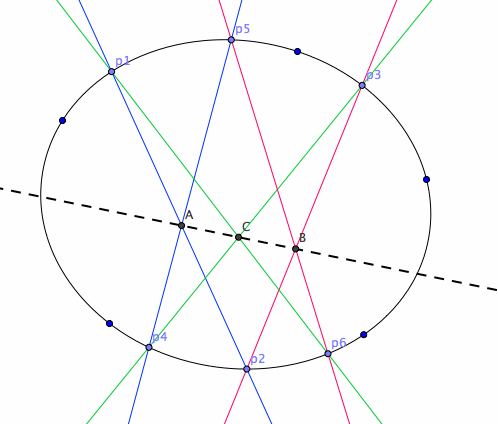Pascal's theorem: The six vertices of a hexagram lie on a conic if and only if the points of intersection of the three pairs of opposite sides lie on a line. Pascal's theorem

Explanation: Pick any six points on a conic (any of hyperbola, parabola, or ellipse), label them 1 to 6 in any order. Connect consecutively labeled points by lines, i.e. {1,2},{2,3},{3,4},{4,5},{5,6},{6,1}. The three pairs of opposite sides are {{1,2},{4,5}},{{2,3},{5,6}},{{3,4},{6,1}}. Pascals theorem states that the three intersections of the pairs are collinear, and, if they are not collinear, then the 6 points do not all lie on a conics.

### Brianchon's Theorem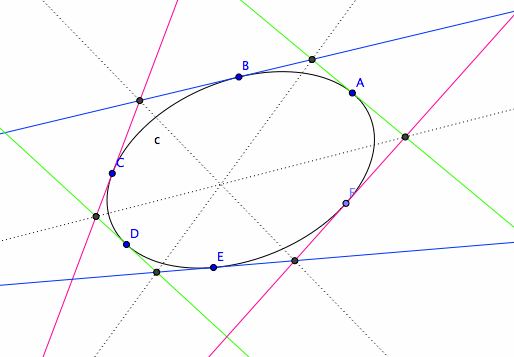Brianchon's theorem: The six sides of a hexagram are tangent to a conic if and only if the lines joining the three pairs of opposite vertices intersect in a point. Brianchon's theorem

Explanation: For Brianchon's theorem, start with six random tangents on a conic. Again label them in any order. Consecutive tangents meet in a total of six points. Connect the three pairs of opposite points, we'll have three lines, and they meet in a point. Note: we might observe that Pascal's theorem isn't true if one or more of the opposite sides are parallel, or that pairs of tangents in Brianchon's theorem may be parallel. Both observations are correct, but in projective geometry parallel lines are said to meet at infinity. This convention reduces wordiness of theorems. From a advanced point of view, points and lines at infinity are parts of basic structure of projective geometry, they are no different than ordinary points or lines.

A shortcut to prove Pascal's therorem is to first prove it for a circle then project the circle to a plane. Since incidence are preserved by projection and circle projects to a conic, thus the theorem is true for any conics.

Given 6 points on a conic, in how many ways can one form a hexagram? See: Number Of Ways To Loop n Points.

### Quadric Surfaces

Equations of polynomials of degree 2 with 2 variables has cross sections that are hyperbola, ellipse, parabola in various ways.EllipsoidParaboloidHyperbolic Paraboloid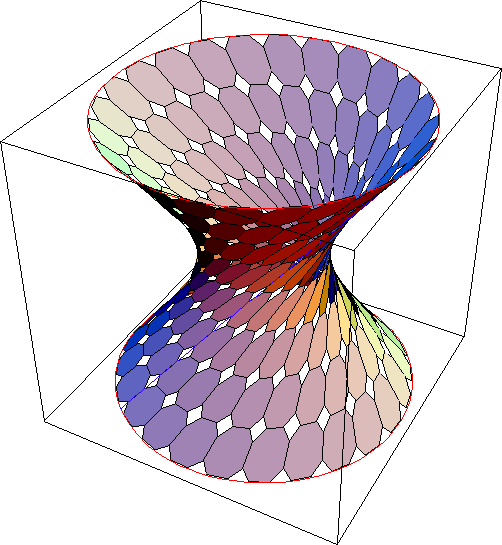Hyperboloid of One SheetHyperboloid of Two Sheet

### Photo of a LampshadeThe is a screenshot from the movie Sex, Lies and Videotape (1989). The image shows a projection of two circles onto a plane. The projection of any circle onto a plane forms a conic section. In this case, it is 2 different hyperbolas.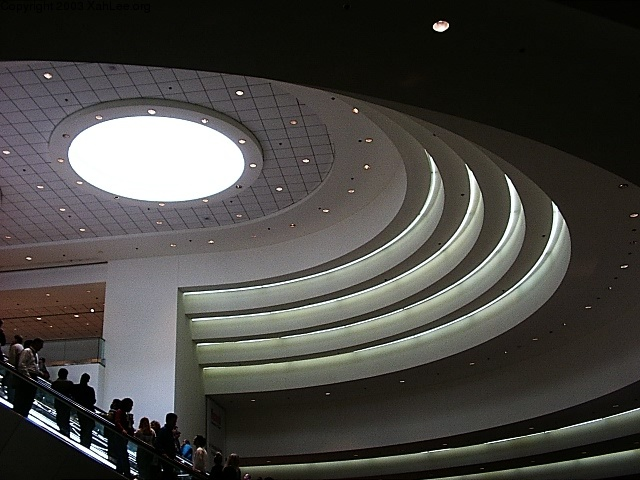Photo of a ceiling light fixture in the shape of circle, at Moscone Center, San Franciosco, USA, 2003. As projected onto the photo, the shape is a ellipse.

## Related Web Sites

Robert Yates: Curves and Their Properties.

If you have a question, put \$5 at patreon and message me.